All submissions of the EM system will be redirected to Online Manuscript Submission System. Authors are requested to submit articles directly to Online Manuscript Submission System of respective journal.

# Bayesian Hierarchical Functional Data Analysis for Healthy Menstrual Cycles

Calvin L Williams* and Trevor Partridge

Department of Mathematical Sciences, Clemson University, Clemson, SC 29634-1907, USA

Corresponding Author:
Calvin L Williams
Department of Mathematical Sciences, lemson University, Clemson, SC 29634- 1907, USA
E-mail:
calvinw@g.clemson.edu

Received: 04/09/2015 Accepted: 09/11/2015 Published: 25/11/2015

Visit for more related articles at Research & Reviews: Journal of Statistics and Mathematical Sciences

## Abstract

In almost every field of study, studying the distribution of a random curve can give needed information. In the field of in vitro fertilization, particular interest is given to modeling the temperature curves of women across their menstrual cycle. Fitting the curve can lead to categorizing cycles as healthy or unhealthy and can help identify the most fertile days of a cycle.

#### Keywords

Hierarchical model, Gibbs sampling, Simulation, Bayesian specification, Menstrual cycles.

#### Introduction

In almost every field of study, studying the distribution of a random curve can give needed information. In the field of in vitro fertilization, particular interest is given to modeling the temperature curves of women across their menstrual cycle. Fitting the curve can lead to categorizing cycles as healthy or unhealthy and can help identify the most fertile days of a cycle. A healthy menstrual cycle consists of three phases, the follicular phase, ovulation, and the luteal phase. During the follicular phase, the basal body temperature (the lowest temperature reached during rest, referred to as bbt for the remainder of this paper) holds constant at a low plateau. During ovulation, the bbt increases until it hits the high plateau. The bbt then holds constant at this high plateau through the luteal phase. At the end of the cycle, the bbt drops back down to the low plateau before menstruation and the cycle repeats . Figure 1 gives a few examples of a healthy menstrual cycle’s bbt levels . Section 2 outlines the general hierarchical model, then gives a parametric modeling of the bbt curves. The end of Section 2 completes the Bayesian specification and specifies the hyperparameters needed for the prior distributions. Section 3 briefly describes how the Gibbs sampling algorithm works and then applies it to model. Section 4 gives a short description of the change-point problem and defines the stopping rule needed in order to identify the two change-points in the model. Section 5 details a simulation of the data and then applies the Gibbs sampling algorithm with intentionally skewed hyperparameters. The results of the simulation are discussed at the end of Section 5. Finally, Section 6 provides a short discussion of the model and conclusions.

#### The Hierarchical Model

First, we will define terms necessary to describing the model. A prior distribution of an unknown quantity X is the probability distribution that would express one’s certainty about X before the data is taken into account. Similarly, a posterior distribution of an unknown quantity X is the probability distribution of that quantity conditional on the observed data. A hyperparameter is parameter of a prior distribution that is not treated as random. For example, consider a random variable X that came from A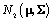distribution, where Σ is known and we can use adistribution to model μ. Then μ is a parameter,is a prior distribution, and μ0 and Σ0 are hyperparameters.

#### The General Model

Letrefer to woman i,jε {1,2,.....ni} refer to cycle j of woman i, and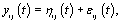refer to day t in cycle j of woman i. These indices will retain these definitions throughout the remainder of the paper. We can establish the following model for bbt curves :where εij is the measurement error and ηij is a smooth function fromto.Since cycles of the same woman are expected to be correlated, we can establish a prior distribution for the distribution of functions for woman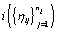call it Gi . However, healthy menstrual cycles will follow a similar pattern among women, so we can establish a prior distribution for the collection of distributions for the different women, call it P. Thus if we assume our error is normally distributed, we have the following model :Basis representation

Now that we have our general model, there are a number of different representations that could be used for ηij. A common strategy is to consider a basis representation :whereare pre-specified basis functions and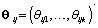are cycle-specific basis coefficients. Sinceis prespecified, our major objective is to model the θijs. The approach we use is to give the θijs a hierarchical normal model :Where Nk(μ,Σ) is the multivariate normal distribution with mean vector μ and covariance matrix Σ. The woman-specific basis coefficients are given by αi, α gives the global mean basis coefficients, and Ω and Ω1 are the precision matrices for the within and between woman variability respectively. So to complete the model, we need to specify the basis functionsand establish the components of θij. We’ll do this in the next section when we consider a parametric hierarchical structure.

Parametric Hierarchical Model

As we discussed in Section 1, bbt levels of a healthy cycle follow a biphasic pattern where the bbt levels start at the low plateau in the follicular phase then quickly rise during ovulation to the high plateau in the luteal phase. Thus we can model ηij (t) as follows :wherewhere θij1 and θij2 indicate the temperature during the follicular phase and the increase in bbt during ovulation respectively for cycle j of woman i. We can further define to be the last day of the follicular phase and rij to be the number of days the bbt rises during ovulation (Figure 2). Thus, if we consider yij (t) as Yij a vector of nij bbt values, we can represent our hierarchical model in matrix form :Thus Xij (t), the tth row of X, corresponds to tth day of cycle j of woman i. So we have defined our pre-specified basis functionand our cycle-specific basis coefficients.

Bayesian Specification

Now we have within (Ω) and between (Ω1) woman variability, the last day of the follicular phase (kij), the number of days during ovulation (rij), the global mean (α), and measurement error variance (σ2) left to model. We’ll use the following equations to model the within and between woman variability:We complete the Bayesian specification with the following priors :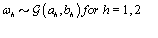where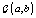is the gamma distribution with shape parameter a and inverse scale parameter b,is the discrete uniform distribution between a and b, and mij is the first day after menstruation. Thus, our pre-specified hyperparameters are α0, Σα, c, d, ah, bh, ah1, and bh2 (for h = 1, 2).A summary of the parameters, hyperparameters, their descriptions, and distributions is given in the appendix.

#### Gibbs Sampling

Our goal is to find the parameters of our model given a set of observations. Since finding the joint distribution is a complicated process, we need to use a different method. A common method to do this is to use a Monte Carlo Markov Chain as an iterative procedure. The specific type of Monte Carlo Markov Chain that we’ll use is called a Gibbs sampler.

#### Definition

Suppose we have random variables X1, X 2,…, X n.

1. Initialization: Letbe given some initial value.

2. Iteration: For 1 ≤ I≤ K, sample x (i)j from the conditional distribution of Xj givenandfor 1 ≤ j ≤ n

This Gibbs sampling procedure is a Markov chain, where the stationary distribution is joint distribution of X1, X 2,…, X n.Thus, for large k, the sampleapproximates the joint distribution of X1, X 2,…, X n. .A blocked Gibbs sampler uses the same steps, except it groups some of the random variables together so that the variables in a block are sampled from their joint distribution conditioned on all other variabes.

Gibbs Sampling Algorithm

Here is the blocked Gibbs sampling algorithm for our model after we’ve given the parameters some initial value :

1. Cycle-specific coefficients: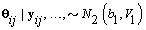2. Women-specific means: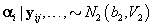3. Global mean:4. Components of Ω( h = 1,2):5. Components of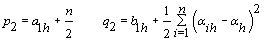6. Error variance:7. Last day of follicular phase: Use the change-point stopping rule for kij (see Section 4).

8. Ovulation period: Use the change-point stopping rule for rij (see Section 4).

So if we use the blocked Gibbs sampling steps to update the unknown parameters from their conditional distributions, then after a large number of iterations, we’ll be sampling from the joint distribution of all the unknown parameters.

#### Change-Point Problem

Now we need to consider kij and rij since these are specific points (called change-points) in the bbt curve where the shape of the curve changes. The process for finding a change-point P is intuitive. Given a set of observations.we’ll find the probability that p ≤ m given ym . We want this probability to be higher than some high threshold Q. Thus we have a “stopping rule" :

Terminate at m if and only ifStarting with m = 1, the first time that the probability is greater than Q is our change-point P.

#### Finding kij

First we wish to find kij assuming that the other parameters are known. Let Xk be the first m rows of the matrix Xij established above but with kij = k. Thus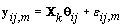. Let {πk} be the prior distribution for kij,π(.) be the density function of θij, and f(.) be the density function of the data yij,m. We now need to find the posterior distribution of kij in order to find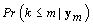for our stopping rule.

Letbe the change-point likelihood. Then implementing Bayes’ theorem, we get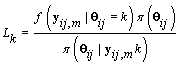Since Lk is not a function θij we may replace θij with 0. Alsodoes not depend on k, so Lk is proportional in k toThe posterior distribution of θij is N2k,Vk) whereNote that this is the same posterior distribution found for θij found in Section 3, but with the altered matrix XK . Using this posterior distribution for θij , we find thatSince we need to find, we’ll first find.From applying Bayes’ theorem to, we getSince LK is proportional in k to WK, then we can substitute Wk in for LK , resulting inThese probabilities are easy to compute as the data becomes available. When k ≥ m,So XK does not depend on k (and hence WK = WM). Thus, our stopping rule is to terminate at m if and only ifOnce we hit our stopping rule , let kij =m.

Finding rij

We can apply the same approach to finding rij by using a transformation to examine the data “backwards", i.e. consider the last day first, and proceed through the cycle in reverse order. So our new reversed data isLetbe our transformation matrix. ThenThus if we let Xr* correspond to the first m rows of the above system, thenThis transformation lets us use the same process that we used to find kij. Let {πr } be the prior distribution for r (the second changepoint). The new posterior distribution for θ*ij is N2r,Vr), whereNote that sincethus giving a similar posterior distribution found in Section 3 for θij. Using this posterior distribution for *ijwe find thatThus, our stopping rule is to terminate at m if and only if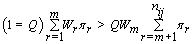Once we hit our stopping rule, r =nij-m (since we transformed our data). Since rij is the number of days between kij and r, then rij = r –kij

#### Data simulation

Now that the method has been described, we’ll introduce a simulation in order to illustrate the method. The simulated data consisted of 20 women, each with 10 cycles, so we had a total of 200 cycles. Each cycle had a length of 30 days . We arbitrarily assigned the global mean and covariance matrices to beEach woman specific mean was generated as per the model discussed in Section 2, soWe then generated kij and rij for each cycle according to.Finally, we generated the cycle specific coefficients according toand the measurement error terms according to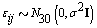where σ 2= 0.01. Now that we generated all the parameters needed for our model, we generated the bbt values according to#### Initialization

In order to run the algorithm, we need to specify the hyperparameters (our initial guesses). In order to account for error, we intentionally perturb the hyperparameters away from the actual parameters established in Data simulation Section So we set our hyperparameters to beThese hyperparamters are used in the Gibbs sampling steps outlined in Change-point probleam section. But before we can implement the Gibbs sampling algorithm, we need to initialize our unknowns from their prior distributions using the hyperparameters, as seen below:We also need to initialize the αis from their prior distributions since the first Gibbs sampling step is finding the posterior distribution of θij conditional on αi . So we’ll generate αi values from the prior distributionThe final step for initialization was to set the kij and rij values all to a fixed value of 7.

#### Running the algorithm

Now that initialization is completed, we can run the algorithm. We ran the algorithm for a total of 6,000 iterations with a 1,000 iteration burn-in. To make sure that the parameters were converging, we performed traceplots of a number of the variables. Figures 3 and 4 show the traceplots for α11 and α12 respectively.

Tables 1 and 2 summarize the results from estimating the parameters in our model. The cycle specific parameters (kij, rij,θij1 and θij2) are summaries over all cycles, while the women specific parameters (αi1 and αi2) are summaries over all women. As evidenced by the tables, the distribution of these parameters was well estimated. Figures 5 and 6 show graphs of the true and estimated distributions for two example cycles.

#### Conclusion

So, we can see that the blocked Gibbs sampling algorithm was an accurate method for estimating the various parameters of our hierarchical model. Most importantly, the change-point analysis gave accurate estimates for the last day of the follicular stage and the number of days that the temperature rises during ovulation (Appendix). These two figures are critical for determining the most fertile period of the menstrual cycle. A natural extension of this topic would be to model unhealthy cycles using a nonparametric approach. Combining the parametric and nonparametric models would allow for broader analysis and more unusual data sets.